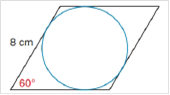Chapter 8.4, Problem 47E### Elementary Geometry for College St...

6th Edition
Daniel C. Alexander + 1 other
ISBN: 9781285195698

#### Solutions

Chapter
Section### Elementary Geometry for College St...

6th Edition
Daniel C. Alexander + 1 other
ISBN: 9781285195698
Textbook Problem
1 views

# Given that the length of each side of a rhombus is 8 cm and that an interior angle measures 60°. Find the area of the inscribed circle.To determine

To Find:

The area of the circle inscribed in a rhombus.

Explanation

The side length of the rhombus is 8 cm.

The angle of the rhombus is 60°.

The diagonals of the rhombus AC and BD bisect each other with right angles at the center of the circle.

The diagonals divide the rhombus in to four 30-60-90 triangles.

In the triangle AOB, We know AB = 8.

In 30-60-90 triangles, the side length ratio is 1:2:3.

Therefore, the length of OB = 4 and OA is 43.

Now, draw a perpendicular OP (radius of the circle) to the side AB of rhombus.

It forms a new 30-60-90 right angle triangle AOP.

Now, we know one side OA is 43

### Still sussing out bartleby?

Check out a sample textbook solution.

See a sample solution

#### The Solution to Your Study Problems

Bartleby provides explanations to thousands of textbook problems written by our experts, many with advanced degrees!

Get Started

#### Solve the equations in Exercises 126. x4x+1xx1=0

Finite Mathematics and Applied Calculus (MindTap Course List)

#### [(13)2]3

Applied Calculus for the Managerial, Life, and Social Sciences: A Brief Approach

#### True or False: f(a)=lima0f(a+h)f(a)h.

Study Guide for Stewart's Single Variable Calculus: Early Transcendentals, 8th